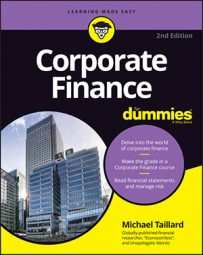##### Corporate Finance For DummiesLong-term assets are those assets that will take more than one year to turn into cash or that are otherwise not intended to be sold yet (but can be sold, if necessary). The long-term assets section of the balance sheet includes three main categories.

## Investments category of long-term assets

Long-term investments typically include equities and debt investments held by the company for financial gain, for gaining control over another company, or in funds such as pensions. It can also include facilities or equipment intended for lease or rent. In any case, all the investments in this section are meant to be held for more than one year.

Note: Sometimes a company lists its bond investments as notes receivables, which are reported sort of like accounts receivables, except with the expectations of receiving payments in the long term.

## Property, plant, and equipment category of long-term assets

The property, plant, and equipment (PPE) category includes nearly every major physical asset a company has that it will use for more than one year. Buildings, machinery, land, major furniture, computer equipment, company vehicles, and even construction-in-progress projects all qualify as PPE. Basically, if you can touch it and plan to use it for more than a single year, it contributes to the value of PPE.

## Depreciation of long-term assets

The long-term physical assets included in PPE don’t last forever. With age and usage, every long-term physical asset is subject to depreciation, or a decrease in value. Different companies measure depreciation in different ways, but regardless of the manner in which a company measures depreciation, the total shows up on the balance sheet as a subtraction from the total value of PPE.

A company may choose to leave out the gross PPE line because it doesn’t really contribute anything to the value of the total assets (and because you can calculate it easily, given the other information listed).

What follows are two of the most common methods for calculating depreciation.

### Straight-line and unit-of-production depreciation

The easiest type of depreciation to use is called straight-line depreciation. Straight-line depreciation is cumulative, meaning that if you report a value in depreciation for a piece of equipment one year, that same amount gets added to the next year’s depreciation, and so on until you get rid of the equipment or its value drops to 0.

For example, if you buy a piece of equipment for \$100 and each year it has a depreciation of \$25, then you’d report \$25 of accumulated depreciation the first year and \$50 of accumulated depreciation the next year, while PPE value would go from \$100 the first year to \$50 the next.

To calculate straight-line depreciation, all you do is start with the original purchase price of the equipment, subtract the amount you think you can sell it for as scrap, and then divide that number by the total number of years that you estimate the equipment will be functional. The answer you get is the amount of depreciation you need to apply each year.

So a piece of equipment bought for \$110 that lasts four years and can be sold as scrap for \$10 has a depreciation of \$25 each year.

A similar type of depreciation, called unit-of-production depreciation, replaces years of usage with an estimated total number of units that the equipment can produce over its lifetime. You calculate the depreciation each year by using the number of units produced that year.

### Sum of years depreciation

The sum of years method for calculating depreciation applies a greater value loss at the start of the equipment’s life and slowly decreases the value loss each year. This method allows companies to take into account the marginally decreasing loss of value that most purchases go through.

Imagine that you’re buying a new car. Unless you get into an accident or otherwise damage the vehicle, the car itself will never lose as much value during its lifetime as it does in the first year. By the time the car is 10 years old, it will have lost most of its value, but it won’t be losing its value as quickly each year.

To calculate the depreciation each year by using the sum of years method, you divide the remaining number of years of life the equipment has left, n, by the sum of the integers 1 through n, and then multiply the answer by the cost of the equipment minus salvage cost. Here’s what that looks like in equation form:

(n / [Σi1 through n]) x (Cost – Salvage value) = Sum of years depreciation

So if you purchase a piece of equipment for \$100,000 and it’s supposed to last for five years with a salvage value of \$10,000, then the first year’s depreciation would look like this:

(5 / [5+4+3+2+1]) x (\$100,000 – \$10,000) = \$30,000

The first year represents 5/15 of the total depreciation that the equipment will go through. The next year is 4/15 (in this case, \$24,000), the next year is 3/15, and so on.